Next: 2.5 State set Up: 2. Choices in the Previous: 2.3 Boundary conditions   Contents

# 2.4 Initial conditions

Not only the boundary conditions need to be specified, but also the initial condition. In most cases the initial condition determines very much the subsequent evolution. Initial conditions can be very special (constructed), or they can be generated randomly.

One important consideration in the generation of initial conditions is that many cellular automata rules conserve some quantities. This can be for example the total number of particles, the total momentum, or energy. It can also be some spurious conserved quantities, i.e., quantities that are not conserved in the system to be modeled, e.g., the number of particles in one row/column. In generating initial conditions, care must be taken that the intended value for the conserved quantities is reached (especially with random initial conditions), and that the spurious conserved quantities do not generate undesired effects.

We have already seen examples of special constructed initial conditions for the example automaton: In Figurewe started with one excited cell (state=2) in a background of quiescent cells (state=0). In Figure 2.3 we started with half a row of excited (state=2) cells, followed by half a row of recovering (state=1) cells in a background of quiescent cells (state=0). This special initial condition, which is constructed to represent a wavefront that is cut off and placed in quiescent medium, leads to the development of a spiral wave.

Now we show the evolution from random initial conditions: If we start with a proportion of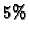cells in state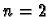, and all others in state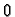, we see in Figure 2.14 that the evolution leads to expanding waves around each seed, which annihilate each other when they meet.

Applet 2.14: Example automaton with random initial conditions (2-cells).

If we start with a proportion ofcells in state, anothercells in state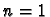, and all others in state, we see in Figure 2.15 a similar evolution. The only difference appears for cases where a cell with value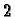and a cell with value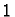lie next to each other, for in this case a center emitting periodic waves is created (two centers in lower right Figure 2.15). In the deterministic Greenberg-Hastings automaton, the number of spiral waves or spiral wave pairs is a conserved quantity.

Applet 2.15: Example automaton with random initial conditions (2-cells and1-cells).Next: 2.5 State set Up: 2. Choices in the Previous: 2.3 Boundary conditions   Contents
Jörg R. Weimar